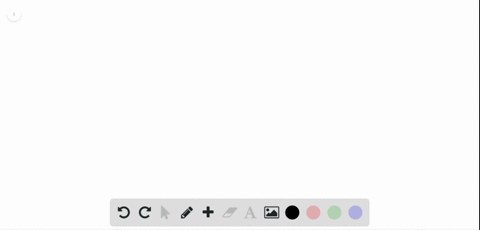### Complete Table 4.8.

04:03
Problem 96Case Western Reserve University
Problem 95

An atom's nucleus has 92 protons and its mass number is
235. How many neutrons are in the nucleus? What is the
name of the atom?

The element is Uranium.

## Discussion

You must be signed in to discuss.

## Video Transcript

by looking at the mass number 235 we can calculate that this element is uranium. Now, in order to get the number of neutrons, as we were given, Rose mass number and protons is we just need to subtract the protons 92 with the mass number 2 35 which gives us the total number of neutrons off the difference, which is 1 43